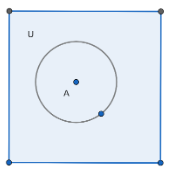QuestionAnswers

# If U is the universal set and $A\subset U$ then fill in the blanks:(a). $A\cup A'=........$ (b). $A\cap A'=.........$ (c). $\phi '\cap A=........$ (d). $U'\cap A=........$Hint: First we will use the Venn diagram and we will solve this question by first understanding the meaning of complement of a set, and then what is union and intersection of sets and then we will find the value of all the options.

Let’s first write the definition of some terms,
Universal set: The set containing all objects or elements and of which all other sets are subsets.
Complement of a set: Complement of a set A, denoted by A c, is the set of all elements that belongs to the universal set but does not belong to set A.
Union: The union (denoted by $\cup$ ) of a collection of sets is the set of all elements in the collection. It is one of the fundamental operations through which sets can be combined and related to each other.
Intersection: The intersection of two sets has only the elements common to both sets. If an element is in just one set it is not part of the intersection. The symbol is an upside down $\cap$ .Let’s start solving each part one by one.
For (a):
$A\cup A'$ covers the whole universal set by the given diagram and definition it is clear.
Hence, $A\cup A'$ = U.
Now we will solve part (b):
Now $A\cap A'$ will be null set by the definition of intersection and complement of the set and from the Venn diagram also we can see that.
Hence,
$A\cap A'=\phi$
Let’s solve part (c):
Now $\phi '$ will be a universal set U.
Now from the given diagram we can see that,
$\phi '\cap A$ will be equal to A.
Hence, $\phi '\cap A$ = A.
For part (d):
Now $U'$ will be null set $\phi$ .
Now from the given diagram we can see that,
$U'\cap A$ will be equal to $\phi$.
Hence, $U'\cap A$= $\phi$.
Hence, we have found all the values.

Note: One can also solve this question by taking some values of the set U and A, and then can find all the values of the given option easily. The definition that we have used should be kept in mind.
View Notes
What is Set, Types of Sets and Their Symbols?What Happens if the Earth Stops Rotating?What is the Difference Between Trade and Commerce?The Difference Between an Animal that is A Regulator and One that is A ConformerCBSE Class 11 Maths FormulasWhat is the Cell Envelope?What is the Placebo EffectWhat is the full form of phd?CBSE Class 6 Maths Chapter 11 - Algebra FormulasCBSE Class 11 Maths Chapter 16 - Probability FormulasImportant Questions for CBSE Class 11 Maths Chapter 1 - SetsImportant Questions for CBSE Class 11 Maths Chapter 7 - Permutations and CombinationsImportant Questions for CBSE Class 11 Maths Chapter 2 - Relations and FunctionsImportant Questions for CBSE Class 7 Maths Chapter 11 - Perimeter and AreaImportant Questions for CBSE Class 11 Maths Chapter 13 - Limits and DerivativesImportant Questions for CBSE Class 11 Chemistry Chapter 11 - The p-Block ElementsImportant Questions for CBSE Class 11 MathsImportant Questions for CBSE Class 11 English Woven Chapter 6 - The StoryImportant Questions for CBSE Class 11 Maths Chapter 11 - Conic SectionsImportant Questions for CBSE Class 11 Chemistry Chapter 10 - The s-Block ElementsMaths Question Paper for CBSE Class 12 - 2016 Set 1 CMaths Question Paper for CBSE Class 12 - 2016 Set 1 NMaths Question Paper for CBSE Class 12 - 2016 Set 1 EMaths Question Paper for CBSE Class 12 - 2016 Set 1 SChemistry Question Paper for CBSE Class 12 - 2016 Set 1 EChemistry Question Paper for CBSE Class 12 - 2016 Set 1 SChemistry Question Paper for CBSE Class 12 - 2016 Set 1 NChemistry Question Paper for CBSE Class 12 - 2016 Set 1 CPhysics Question Paper for CBSE Class 12 - 2016 Set 1 CPrevious Year Question Paper for CBSE Class 12 Physics - 2016 Set 1 SNCERT Solutions for Class 11 Maths Chapter 1NCERT Solutions for Class 9 English Beehive Chapter 11 - If I Were YouNCERT Solutions for Class 11 Maths Chapter 1 Sets in HindiNCERT Solutions Class 11 English Woven Words Poem Chapter 5 The World is too Much With UsRD Sharma Class 11 Maths Solutions Chapter 23 - The Straight LinesRD Sharma Class 11 Maths Solutions Chapter 24 - The CircleRD Sharma Class 11 Maths Solutions Chapter 1 - SetsRelations and Functions NCERT Solutions - Class 11 MathsNCERT Exemplar for Class 11 Maths Chapter 1 - Sets (Book Solutions)NCERT Solutions for Class 11 Maths Chapter 2 Relations and Functions in Hindi